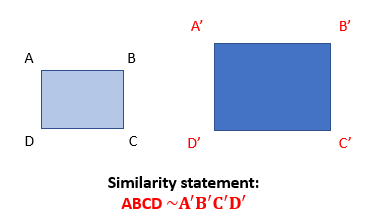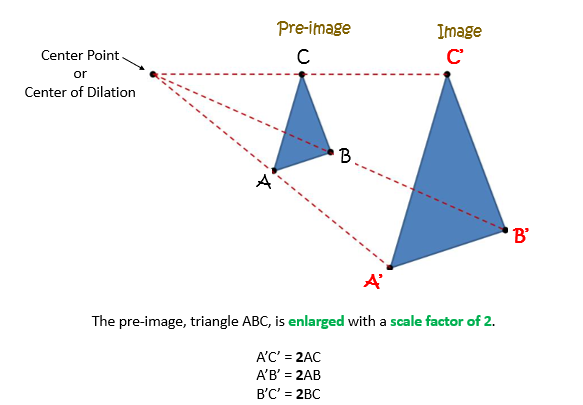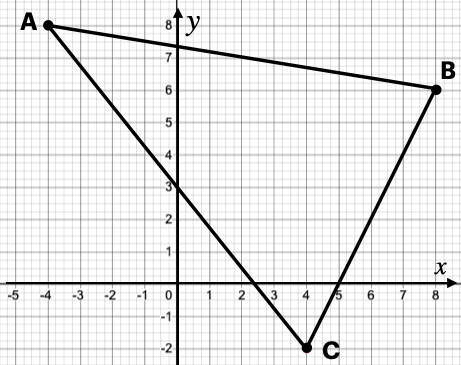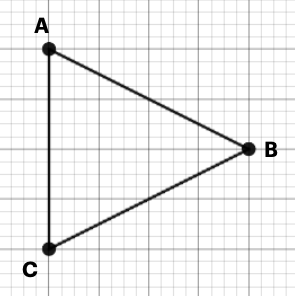## Introduction

• To transform a figure (shape) means changing the size, location, and direction it faces.
• The figure before the transformation is called the pre-image
• The figure after the transformation is called the image
• If the pre-image is labeled as A, then the image would be labeled as A' (pronounced as A prime).
• There are four different types of transformation.
1. Dilation
2. Reflection
3. Rotation
4. Translation

## Similarity

• The image after the dilation is similar to the pre-image.
• Two images are similar if:
• They are of different sizes with proportional sides.
• All angles are congruent.
• They can be mapped on one another with a series of transformations.
• The symbol for similarity is ∼.## Dilation

• A dilation is a type of transformation that resizes (stretches or shrinks) the original figure.
• A dilation produces an image that is the same shape as the original but different in size.
• Dilation produces similar but not congruent images.
• It enlarges or reduces the size of the original figure (pre-image) with a scale factor
• A dilation needs a center point, also called as the center of dilation, and a scale factor.
• The center of dilation or the center point is a fixed point in the plane.
• The scale factor is a ratio of the corresponding sides in a figure.• A scale factor between 0 and 1 means the resulting image will be shrunk/reduced.
• A scale factor > 0 means the resulting image will be enlarged.
• A scale factor of 1 means the pre-image and image are congruent.

## Solved Examples

Question 1: If the scale factor of a dilation centered at the origin is 3, find the image of the point (2, 7).

Solution: If the scale factor is $k=3$, it follows the dilation rule .

Hence, the dilated image of the point (2, 7) will be .

Question 2: $△ABC$ is plotted on the coordinate grid. If the figure were dilated by a scale factor $\frac{5}{2}$ with the origin as the center of dilation, what are the coordinates of the vertices of ${A}^{\text{'}}{B}^{\text{'}}{C}^{\text{'}}$.Solution:

 Pre-image Image after dilation

Question 3: $△{A}^{\text{'}}{B}^{\text{'}}{C}^{\text{'}}$ is the image of $△ABC$ under a dilation with a scale factor of 4 as shown in the figure below. Find the length of segment $\overline{{A}^{\text{'}}{C}^{\text{'}}}$.Solution:

$4=\frac{{A}^{\text{'}}{C}^{\text{'}}}{4}$

## Cheat Sheet

• Look for keywords: Stretch, Enlarge, Reduce, and Shrink.
• In dilation, the figure stretches or shrinks based on a scale factor.
• where k is the scale factor.
• Dilated images are similar but not congruent.

## Blunder Areas

• Differentiate between pre-image (A) and image (A').
• The scale factor in fractions does not always reduce the size of the figure.